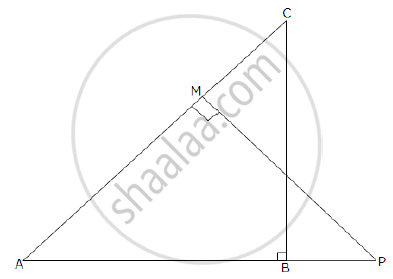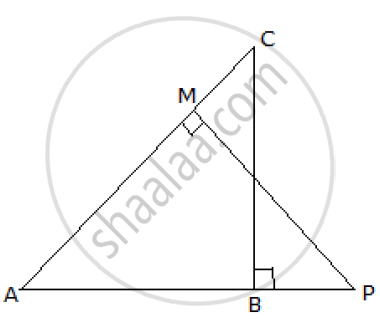Share

# In the Given Figure δAbc and δAmp Are Right Angled at B and M Respectively. Given Ac = 10 Cm, Ap = 15 Cm and Pm = 12 Cm 1) Prove δAbc ~ δAmp 2) Find Ab and Bc. - ICSE Class 10 - Mathematics

#### Question

In the given figure ΔABC and ΔAMP are right angled at B and M respectively. Given AC = 10 cm, AP = 15 cm and PM = 12 cm.1) Prove ΔABC ~ ΔAMP

2) Find AB and BC.

#### Solution

In ABC and AMP

∠ABC = ∠AMP     (each 90°)

∠BAC = ∠PAM     (common)

∴ΔABC ~ ΔAMP   (By AA similarity)

Since the triangles are similar, we have

(AB)/(AM) = (BC)/(MP) = (AC)/(AP)

=> (AB)/(AM) = (BC)/(MP) = (AC)/(AP)

=> (AB)/(AM) = (BC)/12 =  10/15

Taking (BC)/12 = 10/15 =>  BC = (12 xx 10)/15 = 8 cm

Now, using Pythagoras theorem in ΔABC

(AB)^2 + (BC)^2 = (AC)^2

=> (AB)^2 = 10^2 - 8^2 = 36

=> AB = 6 cm

Hence AB = 6 cm and BC = 8 cmIs there an error in this question or solution?

#### APPEARS IN

2011-2012 (March) (with solutions)
Question 8.2 | 3.00 marks

#### Video TutorialsVIEW ALL 

Solution In the Given Figure δAbc and δAmp Are Right Angled at B and M Respectively. Given Ac = 10 Cm, Ap = 15 Cm and Pm = 12 Cm 1) Prove δAbc ~ δAmp 2) Find Ab and Bc. Concept: Similarity of Triangles.
S